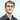# Advent of Code 2019 - Day 4

December 04, 2019

## Day 4 - Secure container

### Part 1 - Whats was that password again…

The elves have lost their password sticky note

It is a six-digit number. The value is within the range given in your puzzle input. Two adjacent digits are the same (like 22 in 122345). Going from left to right, the digits never decrease; they only ever increase or stay the same (like 111123 or 135679).

``````func main() {
input := `357253-892942`
inputs := strings.Split(input, "-")
start, serr := strconv.Atoi(inputs)
finish, ferr := strconv.Atoi(inputs)
meetCriteria := 0
check(serr)
check(ferr)
/**
Loop over input range
**/
for x := start; x <= finish; x++ {
onlyIncreases := true
hasDouble := false
// Loop over each digit in our password
xAsArray := strconv.Itoa(x)
for i := 0; i < len(xAsArray); i++ {
digit, err := strconv.Atoi(string(xAsArray[i]))
check(err)

if i+1 < len(xAsArray) {
check(errL)

hasDouble = true
}

onlyIncreases = false
}
}
}
if onlyIncreases && hasDouble {
meetCriteria++
}
}

fmt.Println(meetCriteria)
}``````

My niave attempt at this first part, simply iterates over all the options from the input, checks for the two main criteria and increments if matching

### Part 2 - No ssstutter

An Elf just remembered one more important detail: the two adjacent matching digits are not part of a larger group of matching digits.

For this I’ve added a record of if we hit a digit with > 2 in a row, if so it is ignored for future loops. Then we keep checking looking for only 2 matches.

``````func main() {
input := `357253-892942`
inputs := strings.Split(input, "-")
start, serr := strconv.Atoi(inputs)
finish, ferr := strconv.Atoi(inputs)
meetCriteria := 0
check(serr)
check(ferr)
/**
Loop over input range
**/
for x := start; x <= finish; x++ {
onlyIncreases := true
hasDouble := false
doubleDigit := -1
// Loop over each digit in our password
xAsArray := strconv.Itoa(x)
for i := 0; i < len(xAsArray); i++ {
digit, err := strconv.Atoi(string(xAsArray[i]))
check(err)

if i+1 < len(xAsArray) {
check(errL)
if i+2 < len(xAsArray) {
check(errL2)

doubleDigit = digit
}
if digit != doubleDigit && digit == oneAhead && digit != twoAhead {
hasDouble = true
}
} else {
if digit != doubleDigit && digit == oneAhead {
hasDouble = true
}
}

onlyIncreases = false
}
}
}
if onlyIncreases && hasDouble {
meetCriteria++
}
}

fmt.Println(meetCriteria)
}``````

### Conclusion

A much nicer problem today then yesterday (much more forgiving), I feel there has to be a cleaner way to approach the more then 2 match problem, but it simply isnt coming to me today.

If you have any feedback on my Go code, I’d be happy to hear it!

Github RepoWritten by Daniel Betteridge who lives and works in Perth, WA building useful things.